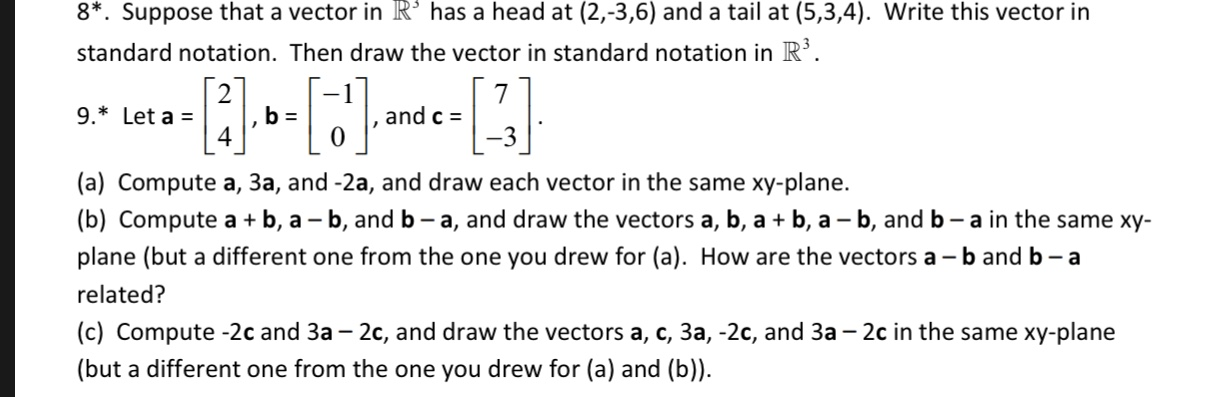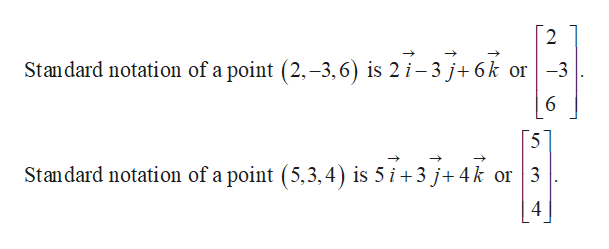8*. Suppose that a vector in R° has a head at (2,-3,6) and a tail at (5,3,4). Write this vector instandard notation. Then draw the vector in standard notation in R7and c =9. Let a4(a) Compute a, 3a, and -2a, and draw each vector in the same xy-plane.|(b) Compute aplane (but a different one from the one you drew for (a). How are the vectors a -b and b - a-b,-b, and b - a, and draw the vectors a, b, a b, a - b, and b - a in the same xy-arelated?(c) Compute -2c and 3a - 2c, and draw the vectors a, c, 3a, -2c, and 3a - 2c in the same xy-plane(but a different one from the one you drew for (a) and (b))

Questionhelp_outlineImage Transcriptionclose8*. Suppose that a vector in R° has a head at (2,-3,6) and a tail at (5,3,4). Write this vector in standard notation. Then draw the vector in standard notation in R 7 and c = 9. Let a 4 (a) Compute a, 3a, and -2a, and draw each vector in the same xy-plane. |(b) Compute a plane (but a different one from the one you drew for (a). How are the vectors a -b and b - a -b, -b, and b - a, and draw the vectors a, b, a b, a - b, and b - a in the same xy- a related? (c) Compute -2c and 3a - 2c, and draw the vectors a, c, 3a, -2c, and 3a - 2c in the same xy-plane (but a different one from the one you drew for (a) and (b)) fullscreen
Step 1

Hey, since there are multiple questions posted, we will answer first question. If you want any specific question to be answered then please submit that question only or specify the question number in your message.

Step 2

The standard notation of a point...help_outlineImage Transcriptionclose2 Standard notation of a point (2,-3,6) is 2 i-3 j+6k or -3 5 Standard notation of a point (5,3,4) is 5 i 3 j+4k or 3 4 fullscreen

Want to see the full answer?

See Solution

Want to see this answer and more?

Our solutions are written by experts, many with advanced degrees, and available 24/7

See Solution
Tagged in

Calculus